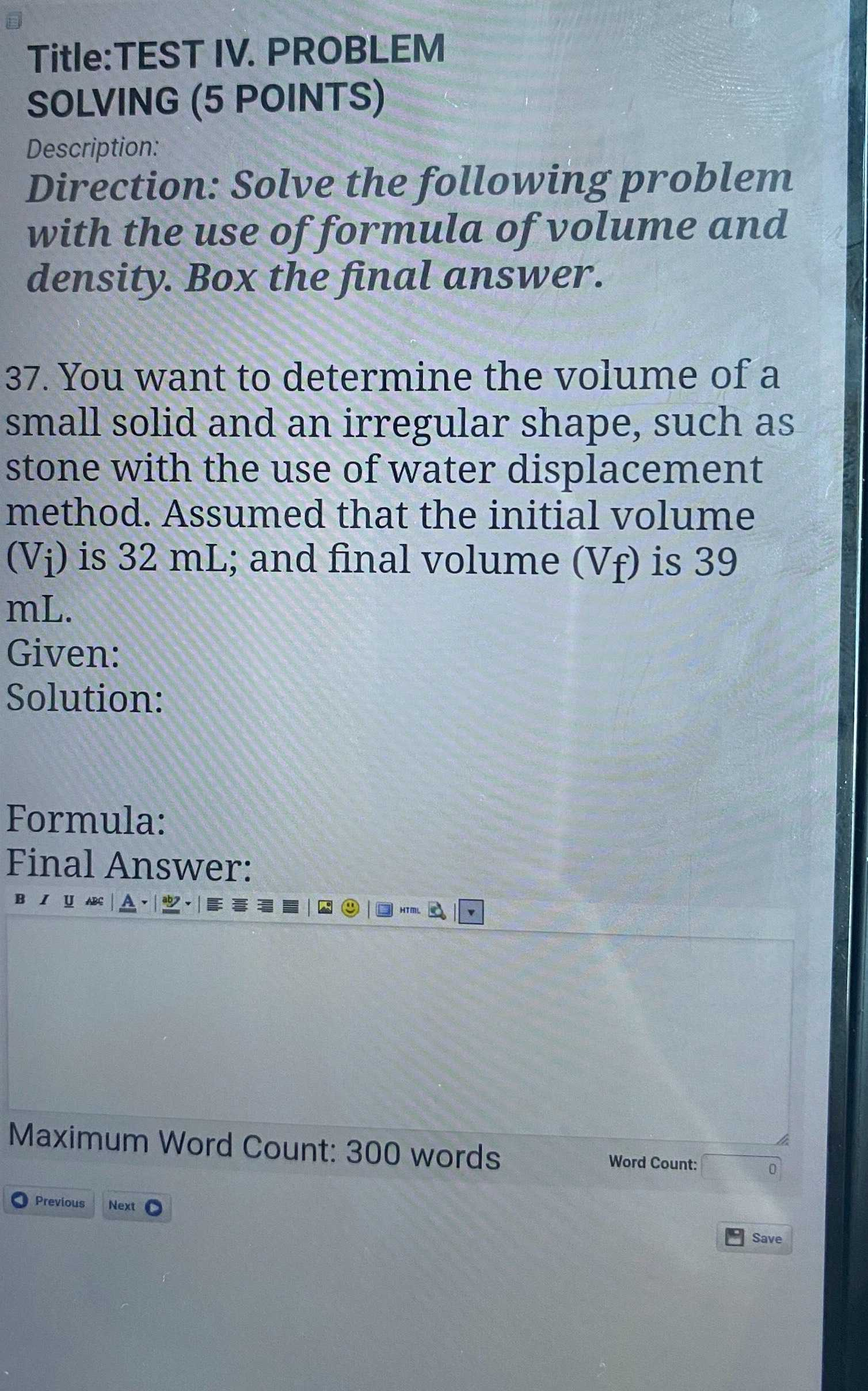### ¿Todavía tienes preguntas de matemáticas?

Pregunte a nuestros tutores expertos
Algebra
Pregunta37. You want to determine the volume of a small solid and an irregular shape, such as stone with the use of water displacement method. Assumed that the initial volume $$( V _ { i } )$$ is $$32 mL$$ ; and final volume $$( V _ { f } )$$ is $$39$$ mL.

Given:

Solution:

Formula: